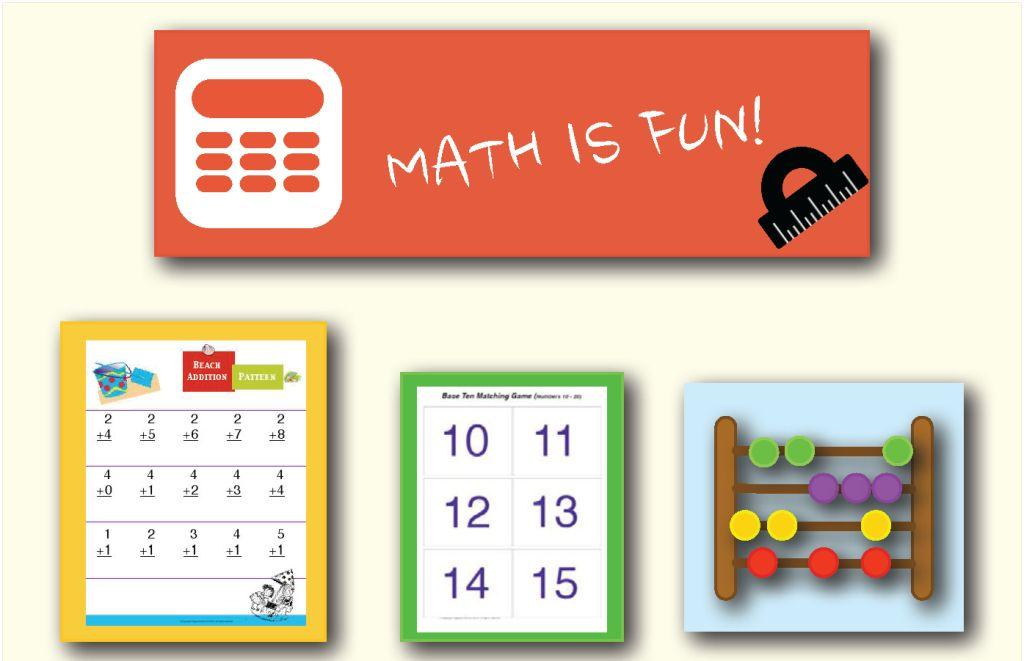# First Grade : Free Math Worksheets

Expand the number sense to counting, comparing, adding, and subtracting numbers. Relate fractions to reality. Learn bar graphs and venn diagrams. Read calendar and clock. Dive deeper into 2-D/3-D shapes and symmetry.

Here is the list of all the topics that students learn in this grade. There are some sample worksheets below each section to provide a sense of what to expect. Each section has some free worksheets too. Please subscribe to access the whole content in its best form.We are aligned to common core standards. Click the button below to the see the curriculum.
Common Core

Click on any topic to view, print, or download the worksheets. All of our worksheets are free for non-commercial and personal use.

Featured Content

Number Line Subtraction

Count back on the number line to solve each subtraction problem.

Category:  Subtraction     Subtraction Strategies